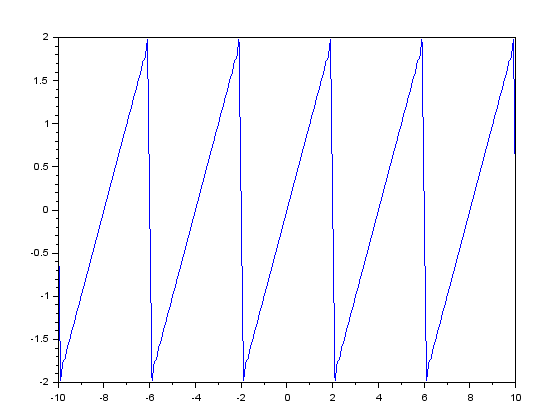# Calculating Fourier Series and plotting it -SCILAB

In the last post I showed you guys how to calculate Fourier Coefficients for a given function defined in the range, [-l,l].

We can also use the Fourier Coefficients to calculate the Fourier Series and then Plot the FS Approximation and compare it to the original function.

I have used the same code as before and just added a few more lines of code.

If you haven’t read the last post which is being continued here then I recommend you read that first here.

Code:

```funcprot(0);
function [a0,A,B]=fourierplot(l,n,f)
clf();
a0=1/l*intg(-l,l,f,.000000001);
for i=1:n
function b=f1(x,f)
b=f(x)*cos(i*%pi*x/l);
endfunction
function c=f2(x,f)
c=f(x)*sin(i*%pi*x/l);
endfunction
A(i)=1/l*intg(-l,l,f1,.000000001);
B(i)=1/l*intg(-l,l,f2,.000000001);
end
function series=solution(x)
series=a0/2;
for i=1:n
series=series+A(i)*cos(i*%pi*x/l)+B(i)*sin(i*%pi*x/l);
end
endfunction
x=-5*l:0.1:5*l;
plot(x,solution(x));
endfunction

```

As you can notice, the code is almost the same as before, except that I have changed the name of the function from ‘fourier’ to ‘fourierplot’. And in the previous post I had only calculated the Fourier Coefficients, a0, A, B, but this time I am using those to calculate the Fourier Series, through the function ‘solution’. And finally I have plotted the Fourier Series.

Sample Demo:

```deff('a=f(x)','a=x');
[A0,A,B]=fourierplot(2,100,f)
```

Output:In the above sample code I have defined a function f(x)=x, and then I have called the function fourierplot with arguments l=2, n=100 & the function f. l is the half of the period so the function is periodic with a period of 2l=4 from [-2,2], which is evident from the graph. If the value of n is not large enough then you might not get a very good approximation.

I have created a module in scilab which contains both the above macro, and once installed can be used as an in-built function. You can download it from here: https://atoms.scilab.org/toolboxes/fstools

Tutorial:

Leave your questions/suggestion/corrections in the comments section down below and I’ll get back to you soon.[wpedon id="7041" align="center"]

## 13 thoughts on “Calculating Fourier Series and plotting it -SCILAB”

1. gj sir

2. It’s showing round off error. How to remove that?
I have taken 0.00000000001

1. Hi there,
Are you getting error for larger tolerances as well? Maybe try using a larger tolerance value.

1. Yes I’m getting error for larger tolerance as well.
It’s showing- round of error and try for large tolerance value.. something like this..

1. Which version of Scilab are you using?

1. 6.0.0

2. Okay, found the problem. Turns out, in the newer(6.0) version we need to pass another argument that is the relative error.
I wrote the code with some older version, therefore it worked for me.
You can try the following code:

```funcprot(0);
function [a0,A,B]=fourierplot(l,n,f)
clf();
a0=1/l*intg(-l,l,f,.000000001,0.00001);
for i=1:n
function b=f1(x,f)
b=f(x)*cos(i*%pi*x/l);
endfunction
function c=f2(x,f)
c=f(x)*sin(i*%pi*x/l);
endfunction
A(i)=1/l*intg(-l,l,f1,.000000001,0.00001);
B(i)=1/l*intg(-l,l,f2,.000000001,0.00001);
end
function series=solution(x)
series=a0/2;
for i=1:n
series=series+A(i)*cos(i*%pi*x/l)+B(i)*sin(i*%pi*x/l);
end
endfunction
x=-5*l:0.1:5*l;
plot(x,solution(x));
endfunction

```
3. Ok
Thank you so much

3. meaning of funcprot(0)

4. what if the function has two or more values in the interval? For example a rectifier?

5. i am still not getting the graph and solution. the code just gets repeated in the console

1. Maybe the code isn’t compaitble with newer versions of Scilab, as it was written quite some time ago.

6. I AM STILL NOT GETTING THE GRAPH